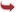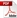International Journal of Scientific and Research Publications

#### IJSRP, Volume 6, Issue 2, February 2016 Edition [ISSN 2250-3153]Determination of First Ionization Energy without Using Shell`S Mechanism of Electrons of All Elements
Nirmalendu Das
Abstract: Electronic configurations of the elements already established in the subject of material science. These were determined by the system of (1s) for Hydrogen (IA group, Period 1), Helium (8A or zero group, Period 1), these numbers added to next to next element with the series of (2s, 2p), (3s, 3p, 3d), (4s, 4p, 4d, 4f), (5s, 5p, 5d, 5f), etc. for other elements up to atomic number 118. There are huge numbers of electrons with respect to atomic numbers. This system is one of the wonder systems in the modern science. Though here in this script I tried to find the 1st ionization energy by using relative numbers which derived from couple system  and periodic numbers, both are new attempted in this field of material science. Relative numbers are very unique, these numbers are able to find the series of Pi , series of ½ , cells functions of mind . There is no relation between number of electrons and relative numbers (r1 and r2), although I observed that, [(r2 – 2) + r1] = Atomic Number of the elements = Z, [r2 > r1 and r2 = (r1 + 2)]. For example, relative number of Hydrogen r2 = 2 and r1 = 1, then [(r2 – 2) + r1] = [(2 – 2) + 1] = 1 = Z. For Helium, r2 = 2, r1 = 2, then [(r2 – 2) + r1] = [(2 – 2) + 2] = 2 = Z. Therefore, relative number is related to atomic number of element. Also, relative numbers of element almost ½ of atomic number.
[VIEW FULL PAPER][DOWNLOAD]

Reference this Research Paper (copy & paste below code):

Nirmalendu Das (2018); Determination of First Ionization Energy without Using Shell`S Mechanism of Electrons of All Elements; Int J Sci Res Publ 6(2) (ISSN: 2250-3153). http://www.ijsrp.org/research-paper-0216.php?rp=P505079